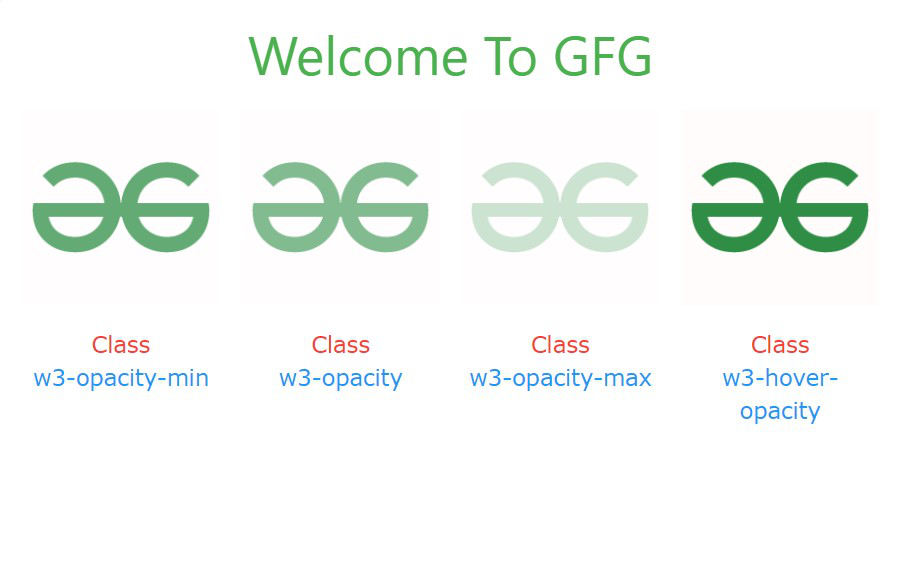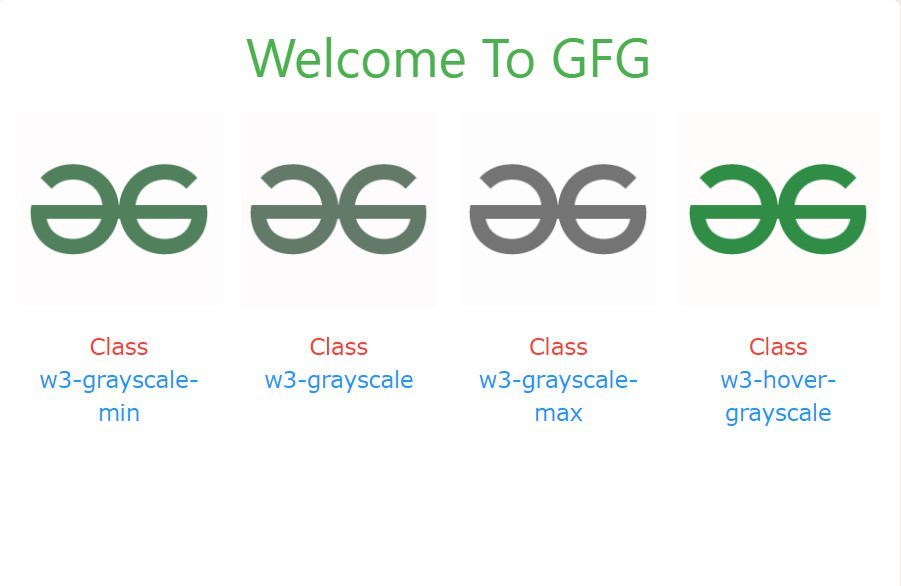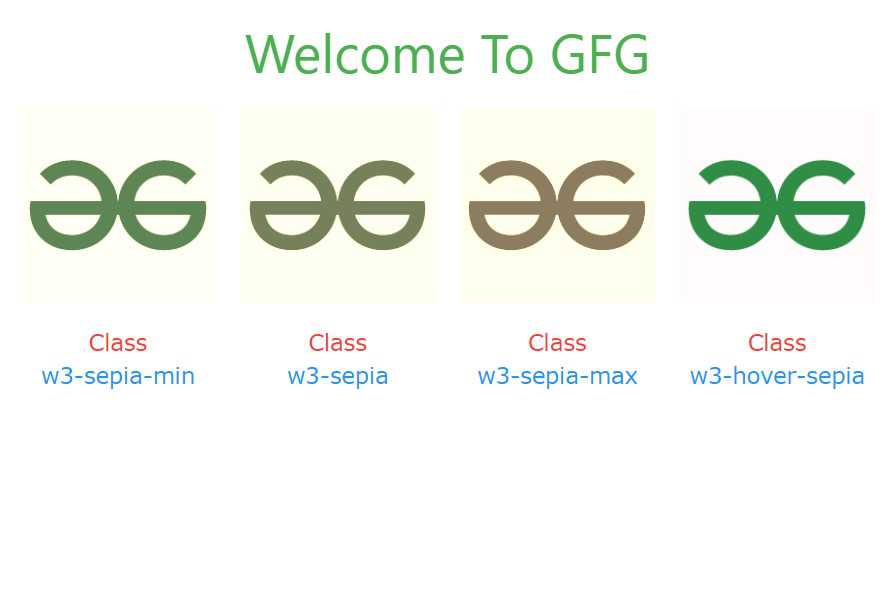Related Articles

# W3.CSS Effects

• Last Updated : 02 Mar, 2021

W3.CSS provides web developers with various effects. They can broadly be classified into three divisions. They are:

• Opacity
• Grayscale
• Sepia

#### Opacity Effects:

There are four opacity effect classes:

Example:

## HTML

 ```<``html``>`` ` `<``head``>`` ` `    ````    ``<``link` `rel``=``"stylesheet"` `href``=``        ``"https://www.w3schools.com/w3css/4/w3.css"``>```` ` `<``body``>``    ````    ````    ``<``div` `class``=``"w3-container w3-center"``>`` ` `        ````        ````        ``<``h2` `class``=``"w3-text-green w3-xxlarge"``>``            ``Welcome To GFG``        ````    ```` ` `    ````    ``<``div` `class``=``"w3-row w3-row-padding w3-center"``>``         ` `        ````        ``<``div` `class``=``"w3-col m3 l3"``>``            ``<``img` `class``=``"w3-image w3-opacity-min"` `                ``src``=``"gfg.png"``>`` ` `            ``<``p``>``                ``<``span` `class``=``"w3-text-red"``>``                    ``Class``                ````                ``<``br``>``                ``<``span` `class``=``"w3-text-blue"``>``                    ``w3-opacity-min``                ````            ```` ` `        ````        ````        ``<``div` `class``=``"w3-col m3 l3"``>``            ``<``img` `class``=``"w3-image w3-opacity"``                ``src``=``"gfg.png"``>`` ` `            ``<``p``>``                ``<``span` `class``=``"w3-text-red"``>``                    ``Class``                ````                ``<``br``>``                ``<``span` `class``=``"w3-text-blue"``>``                    ``w3-opacity``                ````            ```` ` `        ````        ````        ``<``div` `class``=``"w3-col m3 l3"``>``            ``<``img` `class``=``"w3-image w3-opacity-max"` `                ``src``=``"gfg.png"``>`` ` `            ``<``p``>``                ``<``span` `class``=``"w3-text-red"``>``                    ``Class``                ````                ``<``br``>``                ``<``span` `class``=``"w3-text-blue"``>``                    ``w3-opacity-max``                ````            ```` ` `        ````        ````        ``<``div` `class``=``"w3-col m3 l3"``>``            ``<``img` `class``=``"w3-image w3-hover-opacity"` `                    ``src``=``"gfg.png"``>``            ``<``p``>``                ``<``span` `class``=``"w3-text-red"``>``                    ``Class``                ````                ``<``br``>``                ``<``span` `class``=``"w3-text-blue"``>``                    ``w3-hover-opacity``                ````            ```` ` `        ````    `````` ` ``

Output:Grayscale Effects: There are four grayscale effects classes:

Example:

## HTML

 ```<``html``>`` ` `<``head``>`` ` `    ````    ``<``link` `rel``=``"stylesheet"` `href``=``        ``"https://www.w3schools.com/w3css/4/w3.css"``>`` ` ``` ` `<``body``>``    ````    ````    ``<``div` `class``=``"w3-container w3-center"``>`` ` `        ````        ````        ``<``h2` `class``=``"w3-text-green w3-xxlarge"``>``            ``Welcome To GFG``        ````    ```` ` `    ````    ``<``div` `class``=``"w3-row w3-row-padding w3-center"``>``        ````        ``<``div` `class``=``"w3-col m3 l3"``>``            ``<``img` `class``=``"w3-image w3-grayscale-min"` `                ``src``=``"gfg.png"``>`` ` `            ``<``p``>``                ``<``span` `class``=``"w3-text-red"``>Class``                ``<``br``>``                ``<``span` `class``=``"w3-text-blue"``>``                    ``w3-grayscale-min``                ````            ```` ` `        ````        ````        ``<``div` `class``=``"w3-col m3 l3"``>``            ``<``img` `class``=``"w3-image w3-grayscale"` `                ``src``=``"gfg.png"``>`` ` `            ``<``p``>``                ``<``span` `class``=``"w3-text-red"``>Class``                ``<``br``>``                ``<``span` `class``=``"w3-text-blue"``>``                    ``w3-grayscale``                ````            ```` ` `        ````        ````        ``<``div` `class``=``"w3-col m3 l3"``>``            ``<``img` `class``=``"w3-image w3-grayscale-max"` `                ``src``=``"gfg.png"``>``             ` `            ``<``p``>``                ``<``span` `class``=``"w3-text-red"``>Class``                ``<``br``>``                ``<``span` `class``=``"w3-text-blue"``>``                    ``w3-grayscale-max``                ````            ```` ` `        ````        ````        ``<``div` `class``=``"w3-col m3 l3"``>``            ``<``img` `class``=``"w3-image w3-hover-grayscale"` `                ``src``=``"gfg.png"``>`` ` `            ``<``p``>``                ``<``span` `class``=``"w3-text-red"``>Class``                ``<``br``>``                ``<``span` `class``=``"w3-text-blue"``>``                    ``w3-hover-grayscale``                ````            ````        ````    `````` ` ``

Output:Sepia Effects: There are four sepia classes:

Example:

## HTML

 ```<``html``>`` ` `<``head``>`` ` `    ````    ``<``link` `rel``=``"stylesheet"` `href``=``        ``"https://www.w3schools.com/w3css/4/w3.css"``>```` ` `<``body``>``    ````    ````    ``<``div` `class``=``"w3-container w3-center"``>`` ` `        ````        ````        ``<``h2` `class``=``"w3-text-green w3-xxlarge"``>``            ``Welcome To GFG``        ````    ```` ` `    ````    ``<``div` `class``=``"w3-row w3-row-padding w3-center"``>``        ````        ``<``div` `class``=``"w3-col m3 l3"``>``            ``<``img` `class``=``"w3-image w3-sepia-min"` `                ``src``=``"gfg.png"``>`` ` `            ``<``p``>``                ``<``span` `class``=``"w3-text-red"``>Class``                ``<``br``>``                ``<``span` `class``=``"w3-text-blue"``>``                    ``w3-sepia-min``                ````            ```` ` `        ````        ````        ``<``div` `class``=``"w3-col m3 l3"``>``            ``<``img` `class``=``"w3-image w3-sepia"` `                ``src``=``"gfg.png"``>`` ` `            ``<``p``>``                ``<``span` `class``=``"w3-text-red"``>Class``                ``<``br``>``                ``<``span` `class``=``"w3-text-blue"``>``                    ``w3-sepia``                ````            ```` ` `        ````        ````        ``<``div` `class``=``"w3-col m3 l3"``>``            ``<``img` `class``=``"w3-image w3-sepia-max"``                ``src``=``"gfg.png"``>`` ` `            ``<``p``>``                ``<``span` `class``=``"w3-text-red"``>Class``                ``<``br``>``                ``<``span` `class``=``"w3-text-blue"``>``                    ``w3-sepia-max``                ````            ```` ` `        ````        ````        ``<``div` `class``=``"w3-col m3 l3"``>``            ``<``img` `class``=``"w3-image w3-hover-sepia"``                ``src``=``"gfg.png"``>`` ` `            ``<``p``>``                ``<``span` `class``=``"w3-text-red"``>Class``                ``<``br``>``                ``<``span` `class``=``"w3-text-blue"``>``                    ``w3-hover-sepia``                ````            ````        ````    `````` ` ``

Output:My Personal Notes arrow_drop_up